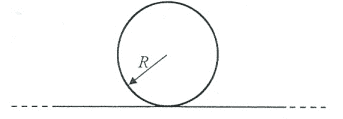# Finding the induced EMF in a solenoid-wire system

Homework Statement:
We have a very big solenoid like the figure below. (it stretches outside and inside of the screen) It has radius ##R## and number of turns per length ##n##. A very large wire of length ##L>>R## is tangent to the solenoid and perpendicular to its axis (without physically connecting to the solenoid). The Current in solenoid is changing with rate ##\alpha = di/dt##. Find the induced emf on the wire. Note that it is a question of Undergrad General Physics and is not meant to be solved with very advanced mathematical techniques.
Relevant Equations:
##d \phi / dt = - \epsilon## ,##\phi = \int B da##
I know that ##B = \mu n I## and ##\phi = B \pi R^2##. So with have ##d\phi / dt = \mu n \alpha \pi R^2##. But I don't know what to do with this? is this the answer? I don't think so but I don't know what to do after this.rude man
Homework Helper
Gold Member
Problem Statement: We have a very big solenoid like the figure below. (it stretches outside and inside of the screen) It has radius ##R## and number of turns per length ##n##. A very large wire of length ##L>>R## is tangent to the solenoid and perpendicular to its axis (without physically connecting to the solenoid). The Current in solenoid is changing with rate ##\alpha = di/dt##. Find the induced emf on the wire. Note that it is a question of Undergrad General Physics and is not meant to be solved with very advanced mathematical techniques.
Relevant Equations: ##d \phi / dt = - \epsilon## ,##\phi = \int B da##

I know that ##B = \mu n I## and ##\phi = B \pi R^2##. So with have ##d\phi / dt = \mu n \alpha \pi R^2##. But I don't know what to do with this? is this the answer? I don't think so but I don't know what to do after this.

View attachment 245176
This one is fun.
My 1st reaction was zero.

But then: think of the solenoid-wire system as a transformer. Then do two Gedankenexperimente a la Einstein:

Setup 1. consider the wire as the primary "winding" and the solenoid (coil) as the secondary. Coil is open. Then you can write the voltage across the coil in 2 ways: in terms of a changing flux and in terms of a changing primary current. In other words, this is just mutual inductance M and you can actually compute M for this setup.

Setup 2: now make the primary the coil and the secondary the wire. The wire is open. You can now write the voltage across the wire in terms of M and the coil current.

A very good problem IMO.

Realizing that it's the same M (not easy to prove BTW) you can now find the voltage across the wire.